Mathematics

# Geometric Sequence

5.5k views

## Table of Contents

 1 Introduction 2 What is a geometric Sequence 3 Applications of geometric Progression in real life 4 First term of GP, Common Ratio 5 nth Term Formula 6 Geometric Sequence Formula 7 Sum of Geometric Sequence 8 GP Graph and Properties 9 Geometric Mean 10 Summary 11 Frequently Asked Questions (FAQs)

21 September 2020

Read time: 6 minutes

## Introduction

In Maths, Geometric Sequence, is called as a Geometric Progression, is a sequence of numbers where each term after the first is found by multiplying the previous one by a fixed, non-zero number called the common ratio.

The common ratio multiplied here to each term to get a next term is a non-zero number. An example of GP is $$2, 4, 8, 16, 32, 64, …,$$ where the common ratio is $$2.$$

Sequence

A Sequence is a set of numbers/things that are in order.

To learn more about Sequences and series please visit

## What is a geometric Sequence?

### Geometric Sequences

In a Geometric Sequence or geometric progression each term is found by multiplying the previous term by a constant.

 Example :

$$1, 2, 4, 8, 16, 32, 64, 128, 256...$$

This sequence has a factor of $$2$$ between each number.

Each term (except the first one) is found by multiplying the previous term by $$2.$$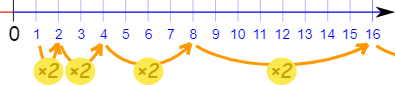Generally, we write a Geometric Sequence like this

$$\left\{ {a,{\rm{ }}ar,{\rm{ }}a{r^2},{\rm{ }}a{r^3},{\rm{ }}...{\rm{ }}} \right\}$$

In the above sequence,

• a is the first term, and

• r is the factorized number between the terms (called the "common ratio")

 Example :

$$\text{{1, 2, 4, 8, 10, 12 ...}}$$

The sequence starts from $$1$$ and doubles each time, so

$$\rm{a} = 1$$ (first term)

$$\rm{r} = 2$$ ("common ratio" between terms is a doubling)

And we get:

\begin{align}\left\{ {\rm{a},{\rm{ ar}},{\rm{ a}}{r^2},{\rm{ }}a{r^3},{\rm{ }}...{\rm{ }}} \right\}&\\\\&= {\rm{ }}\left\{ {1,{\rm{ }}1 \times 2,{\rm{ }}1 \times {2^2},{\rm{ }}1 \times {2^3},{\rm{ }}...{\rm{ }}} \right\}\\\\ &= {\rm{ }}\left\{ {1,{\rm{ }}2,{\rm{ }}4,{\rm{ }}8,{\rm{ }}...} \right\}\end{align}

### Why "Geometric" Sequence?

Because it is increasing the dimensions in geometry: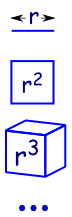A line is $$1$$-dimensional and it has a length of $$\rm{r}.$$ in $$2$$ dimensions a square, it has an area of $$\rm{r}^2$$ in $$3$$ dimensions a cube has volume $$\rm{r}^3$$  And so on………...

## Applications of geometric Progression in real life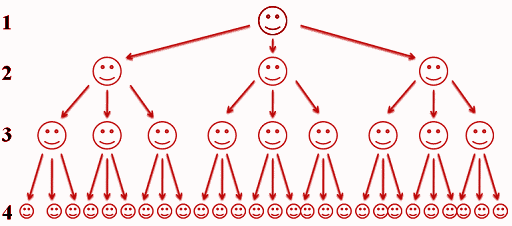In real life, Geometric Progression happens whenever each agent of a system acts independently and is fixed. I am just trying to show that we actually see a lot of sequences and series every day in our daily life.

Will give you some examples these are:

• A population growth in which each people decide not to have another kid based on the current population then population growth each year is geometric.• Each radioactive independently disintegrates so each will have its fixed decay rate so it’s also geometric## First term of Geometric Progression, Common Ratio

### First Term of GP

The general form of Geometric Progression is:

$$\rm{a},{\rm{ ar}},{\rm{ ar}}{^2},{\rm{ ar}}{^3},{\rm{ar }}{^4}, \ldots ,{a_n}$$

Where,

$$a =$$ First term

$$r =$$ common ratio

### Common Ratio of GP

Consider the sequence $$\text{a, ar, ar}^2, \rm{ar}^3,……$$

First term $$= a$$

Second term $$\rm{}= ar$$

Third term $$\rm{}= ar^2$$

Similarly nth term, $$\text{tn = ar}^{n-1}$$

Thus, Common ratio = (Any term)

\begin{align}&= \frac{\rm{t_n}}{\rm{t_{n-1}}} \\\\&= \frac{\rm{(ar^{n – 1})}}{\rm{(ar^{n – 2})}}\\\\&=\text{r}\end{align}

Then, the general term of a Geometric progression/Geometric Sequences given by arn-1 and the general form of a G.P is $$\text{a + ar + ar}^2 + …..$$

For Example: $$\text{r} = \frac{ t2} { t1} = \frac{ar}{a} = r$$

## nth Term Formula

Now, we come to the nth formula of the Geometric Sequence/Geometric Progression.

To find a formula for geometric sequence,

Let a is the first term and r be the common ratio for a Geometric sequence

Then the second term, $$a_2 = \rm{a} \times \rm{r = ar}$$

Third term, $$\rm{a}_3 = a_2 \times r = ar \times r = ar_2$$

Similarly, nth term, $$\rm{}a_n = ar^{n-1}$$

Therefore, the formula to find the nth term of GP is:

 $$\rm{}a_n = ar^{n-1}$$

$$n$$ is the number of terms

Remember :- nth term is the last term of GP.

## Geometric sequence formula

We are using geometric sequence formulas to find out the value of the series, or find out the nth term.

 The Rule

We can calculate any term using the Rule:

 $$\rm{}x_n = ar^{(n-1)}$$

(We are using "$$n-1$$" because $$\rm{ar}^0$$ is for the 1st term)

 Example 1

$$10, 30, 90, 270, 810, 2430, ...$$

The sequence has a factor of 3 between each number.

The values of a and r are:

• a = 10 (the first term)

• r = 3 (the "common ratio")

### The Rule for any term is:

$$\rm{}x_n = 10 \times 3^{(n-1)}$$

So, the 3th term is:

$$\rm{}( x_3 = 10 \times 2^{(3-1)} = 10 \times 2^2 = 10 \times 4 = 40 )$$

A Geometric Sequence also has smaller and smaller values:

$$4, 2, 1, 0.5, 0.25, ...$$

This sequence has a factor of $$0.5$$ between each number.

Its Rule is $$\rm{}x_n = 4 \times (0.5)^{n-1}$$

Geometric sequences can be specified by either of the two types of formulas.

• Recursive Formula

• Explicit Formula

### Recursive Formula

Geometric sequence recursive formula can be used to find many terms by multiplying each previous term to find the next.

We can write a geometric sequence recursive formula in two parts: a statement of the first term along with a statement of the formula relating successive terms.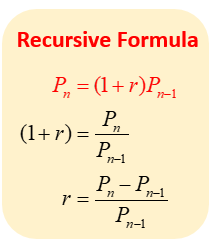$$\rm{}P_n = (1+r) * P_{n-1}$$

Where $$\rm{}r =\frac{ (P_{n} - P_{n-1} )}{ P_{n-1}}$$

 Example 1

$$\rm{r} = 2 a_6 = 11$$. Find $$a_7$$

Solution

Using recursive formula, use a6 to find a7

Hence, $$\rm{}a_6 * r = a_7$$

$$\rm{}a_7 = 2*11 = 22$$

### Explicit Formula for the geometric sequence.

Explicit geometric formulas find many terms using a starting term and exponential growth. So, the explicit formula for the geometric sequence is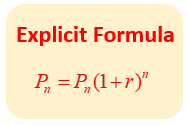$$\rm{}P_n = P_0 * (1+r)^n$$

## Sum of Geometric sequence

To find the sum of GP, we need to see the types of geometric series.

There are two types sum of geometric sequence these are

• Finite sum
• Infinite sum

### Finite sum

To find the sum of a finite geometric series, the formula is,

$$\rm{}Sn = \frac{a_1 (1−r^{n})} {(1−r) , \,r ≠ 1,}$$

where $$n$$ is the number of terms,

$$a_1$$ is the 1st value and r is the common ratio.

\begin{align}&\bf{\text{Sums of Geometric Sequences and Series}}\\\\ &\text{Sum of finite geometric sequence:}\\\\ &\qquad\qquad \qquad S_{n}=\sum_{i=1}^{n} a_{i} r^{i-1}=a_{1}\left(\frac{1-r^{n}}{1-r}\right)\end{align}

### Infinite sum

An infinite geometric sequence is the sum of an infinite geometric sequence.

The general form of the infinite geometric series is $$\rm{}a_1 + a_1r + a_1r_2 + ... ,$$where $$a_1$$ is the first term and $$r$$ is the common ratio. We can find the sum of all finite geometric sequences or finite geometric series.

$$\begin{array}{l}{\text{Sum of infinite geometric series:}}\\\qquad \qquad \qquad \qquad S = \sum\limits_{i = 0}^\infty {{a_i}} {r^i} = \frac{{{a_1}}}{{1 - r}}\\{\text{[Note: If |r|}} \ge {\text{1, the infinite series does not have a sum]}}\end{array}$$

## GP graph

The manners of a geometric sequence depend on the value of the common ratio. If the common ratio is positive, so then the terms will all be the same sign as the initial term. negative, the terms will be possible between positive and negative.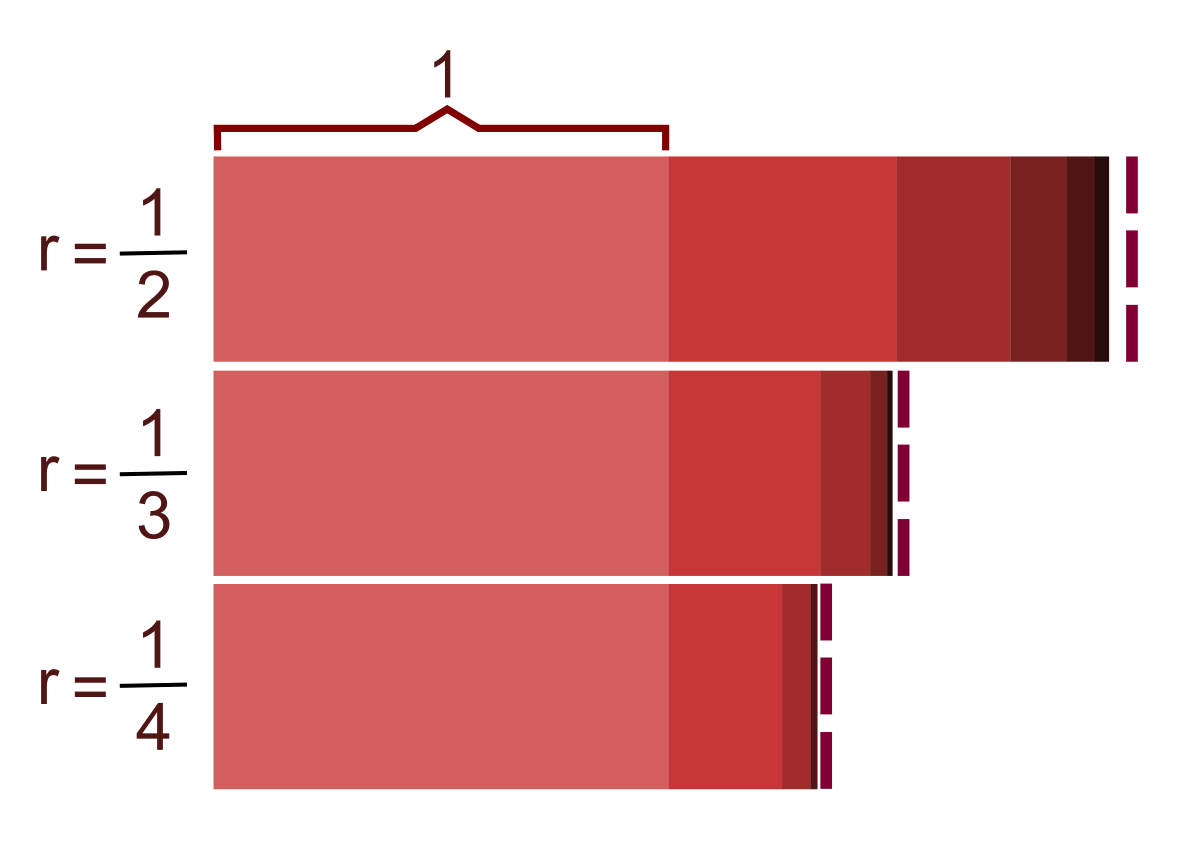## Geometric Mean

In mathematics, the geometric mean is a mean, which specifies the central tendency of a set of numbers by using the multiply of their values.

${\text{Geometric}}\,{\rm{Mean = }}\left[ {\left( {{\rm{1 + }}{{\rm{r}}_{\rm{1}}}} \right){\rm{ \times }}\left( {{\rm{1 + }}{{\rm{r}}_{\rm{2}}}} \right){\rm{x \ldots x}}{{\left( {{\rm{1 + }}{{\rm{r}}_{\rm{n}}}} \right)}^{\frac{{\rm{1}}}{{{\rm{n - 1}}}}}}} \right]$

## Summary

A geometric sequence or progression is a sequence where each term is calculated by multiplying the previous term by a fixed number.

A geometric sequence has:

• a first term, a,
• a common ratio, r.

If the terms of a geometric sequence are added together a geometric series is formed.

There are formulae for calculating the sum of a number of terms of a geometric sequence. an infinite geometric series can tend towards a finite number.

Written by Neha Tyagi, Cuemath Teacher

## Can the numbers in a geometric sequence go down

Yes, if the common ratio is a fraction the terms will decrease.

## What happens in a geometric sequence when the common ratio is negative

Each term will change sign, that is the sequence will oscillate.

e.g. A sequence with a first term of 3 and a common ratio of -2 will be 3, -6, 12, -24, 48, …

Yes

## Geometric vs Arithmetic

Each term of a geometric series is calculated by multiplying the previous term by a fixed amount whereas each term of an arithmetic series is calculated by adding a fixed amount to the previous term.

## When does a geometric sequence have a sum to infinity?

When the common ratio is a positive or negative fraction less than 1.

i.e. -1 < r < 1

GIVE YOUR CHILD THE CUEMATH EDGE
Access Personalised Math learning through interactive worksheets, gamified concepts and grade-wise courses
Learn More About Cuemath